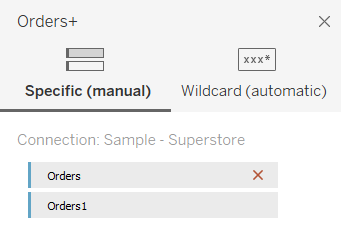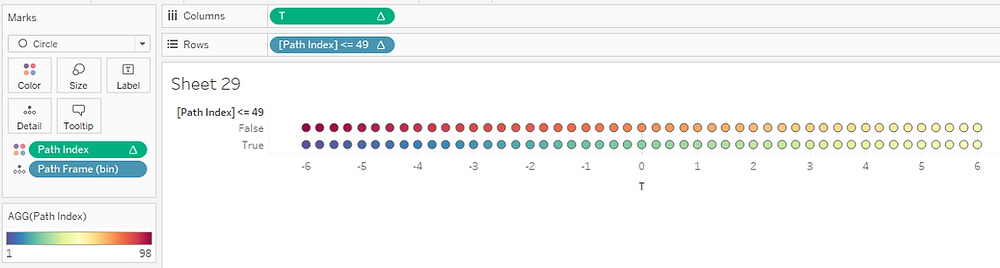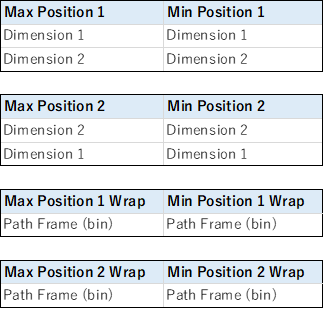# SANKEY DIAGRAM with self-union

Sankey Diagram is a popular and powerful way to show a flow or a relationship between two dimensions, but how to create it is a bit complicated.

As far as I know, originally they prepare an Excel file for a sigmoid-curve, and Ian from The Information Lab shared his idea to create the diagram without any calculations.

Since Ian's idea is using a LOD calculation to allow data to have a dimension for a data densification which is critical for the sigmoid-curve, it requires a column which could work as a record identifier (although the way he introduced was quite amazing and mind-blowing and I loved it).

While above, we also use a self-UNION method for a most of charts using a curve or a connective line, such as a Jump Plot. I thought it could be applied to a Sankey Diagram as well.

The purpose of this blog post is to introduce a way to create a Sankey Diagram with self-UNION method, which just double a data size but it allows us to have a simplicity compared to Ian's way.

The workbook used in this blog post is available in Tableau Public and I used Super SampleStore data.

### Preparation

It starts with self-UNION. You can simply UNION the order sheet.And we create these calculations below.

Chosen Measure (for Sankey polygon size)

SUM([Sales])

Dimension 1

[Region]

Dimension 2

[Category]

Path Frame

IIF([Table Name] = 'Orders', 0, 97)

Path Frame (bin)Then we can have a table like below.

Each combination of the Dimension1 and Dimension2 will turn into each polygon of a Sankey Chart, and the numbers(Chosen Measure) will be used for the size of each polygon.### SIGMOID CURVE CALCULATIONS

In the Sankey Diagram, the Sigmoid Curve is used and we need to prepare some calculation fields.

Path Index (calculated with Path Frame(bin))

INDEX()

T

IF [Path Index] <= 49

THEN (([Path Index] - 1) % 49) / 4 - 6

ELSE - (([Path Index] - 1) % 49) / 4 + 6

END

Sigmoid

1 / (1+EXP(1)^-[T])

Let's start with Path Index. As mentioned earlier, we will use data densification.

By Clicking 'Show Missingvalue' option, we can increase the number of marks in the worksheet.Then, let's set the Path Index column calculated along with Path Frame(bin).The Path Index gives us integers from 1 to 98 according to the Path Frame(bin).

With this integers we calculate T for the Sigmoid curve.Please notice that, for each T value from -6 to 6 in steps of 0.25, they can have two Path Index. This is required since we will create polygon curves that go from left to right.

Conceptually, by connecting the two lines shown below we draw a Sigmoid curve polygon.### SANKEY DIAGRAM CALCULATIONS

So we have seen some foundational calculations and an idea of the Sigmoid curve.

Then let's take a look at calculations to draw the actual diagram.

Sankey Arm Size (using dimention1 and dimension2)

[Chosen Measure] / TOTAL([Chosen Measure])

This defines the size of each polygon, and this simply returns the percent of total.And here is a list of required calculations. They are all Table Calculations and their settings are shown in a table below the list.

Max Position 1

RUNNING_SUM([Sankey Arm Size])

Min Position 1

[Max Position 1] - [Sankey Arm Size]

Max Position 1 Wrap

WINDOW_MIN([Max Position 1])

Min Position 1 Wrap

WINDOW_MIN([Min Position 1])

Max Position 2

RUNNING_SUM([Sankey Arm Size])

Min Position 2

[Max Position 2] - [Sankey Arm Size]

Max Position 2 Wrap

WINDOW_MIN([Max Position 2])

Min Position 2 Wrap

WINDOW_MIN([Min Position 2])

Table Calculation SettingsFor example with Mas Position 1, you can open the calculation field, and modify the settings.Let's take a quick look at the calculations.The Min Position gives where the polygon starts.

For example, if it is the first polygon, then it returns 0. If it's the second, then it returns the Sankey Arm Size of the first.

As you see from the formula, The Max Position gives where the polygon ends.

The Wrap calculations assign the Max/Min Positions on each Path Frame(bin).

Thanks to these calculations, each Path Frame(bin) can have values although originally there is no data for the bins between the start and the end.

Finally, we can have a formula for the Sankey Polygons.

Sankey Polygons

IF [Path Index] > 49

THEN

[Max Position 1 Wrap]

+ ([Max Position 2 Wrap] - [Max Position 1 Wrap])

* [Sigmoid]

ELSE

[Min Position 1 Wrap]

+ ([Min Position 2 Wrap] - [Min Position 1 Wrap])

* [Sigmoid]

END

By putting the dimensions and calculation fields accordingly, we can get the Sankey Diagram.

If you don't get the same output, then please check your table calculation settings (most of the issues should come from this part).### You can also put a margin between the dimensions

Here is an extra bonus. My workbook also includes some calculations to put a margin on the diagram, which can improve visibility if the dimension1 and/or the dimension2 has the proper number of items in them.